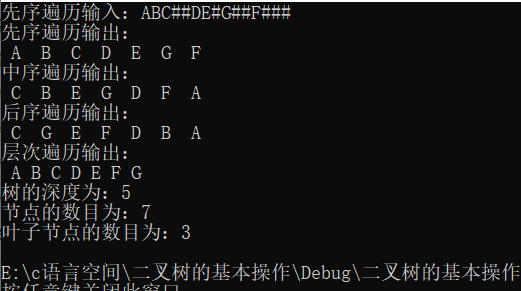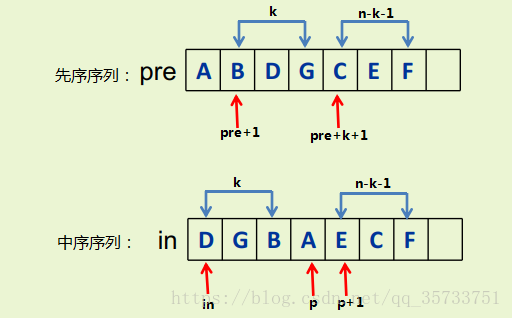• 编写程序，用先序递归遍历法建立二叉树的二叉链表存储结构，然后输出其先序、中序、后序遍历第k个访问结点。二叉树结点数据类型建议选用字符类型且各结点数据域值互不相同；输出用结点数据域的字符表示；求第k个访问...
• //输出先序遍历第k个节点的值 int n =0; void Travel(BTNode *T, int k) {  if(NULL != T)  {  if(++n == k)  {  printf("%d个节点的数据为:%c\n",k,T->data);
//输出先序遍历中第k个节点的值 int n =0; void Travel(BTNode *T, int k) {      if(NULL != T)      {              if(++n == k)              {                     printf("第%d个节点的数据为:%c\n",k,T->data);                     return ;              }                            Travel(T->lchild,k);              Travel(T->rchild,k);                    }
}

//输出中序遍历中第k个节点的值 int n =0; void Travel(BTNode *T, int k) {      if(NULL != T)      {                           Travel(T->lchild,k);

if(++n == k)              {                     printf("第%d个节点的数据为:%c\n",k,T->data);                     return ;              }                            Travel(T->rchild,k);                    }       }

//输出后序遍历中第k个节点的值 int n =0; void Travel(BTNode *T, int k) {      if(NULL != T)      {                                        Travel(T->lchild,k);              Travel(T->rchild,k);

if(++n == k)              {                     printf("第%d个节点的数据为:%c\n",k,T->data);                     return ;              }                    }       }    
展开全文c语言 数据结构 源代码 c
• 文章目录二叉树的特征二叉树的重要性质二叉树的创建二叉树的先序遍历二叉树的中序遍历二叉树的后序遍历二叉树的层序遍历二叉树的深度二叉树的节点数目二叉树叶子节点的数目全部代码测试结果 二叉树的特征 每个节点...


文章目录
二叉树的定义二叉树的特征二叉搜索树平衡二叉树二叉树的重要性质二叉树的创建二叉树的先序遍历二叉树的中序遍历二叉树的后序遍历二叉树的层序遍历二叉树的深度二叉树的节点数目复制二叉树二叉树叶子节点的数目全部代码测试结果

二叉树的定义
一个有穷的节点集合
二叉树的特征
每个节点最多只有两棵子树每棵子树有左右之分，其次序不能颠倒，是有序数
二叉搜索树
定义：一棵二叉树，可以为空；如果不为空，满足以下性质：
非空左子树的所有值小于其根节点的键值非空右子树的所有值大于其根节点的键值左右子树都是搜索二叉树
平衡二叉树
空树或者任一节点左、右子树高度差的绝对值不超过1
二叉树的重要性质
在二叉树的第i层上至多有 2^(i-1)个节点深度为k的二叉树上至多含有2^k-1(k>=1)个节点对任意一棵二叉树，若它含有n0个叶子节点、n2个度为2的节点，则必存在n0 = n2+1具有 n 个结点的完全二叉树的深度为 [ log2n]+1 ([ log2n]：表示以2为底，对n取对数，并向下取整）若对含 n 个结点的完全二叉树从上到下且从左至右进行 1 至 n 的编号，则对完全二叉树中任意一个编号为 i 的结点： (1) 若 i=1，则该结点是二叉树的根，无双亲；否则，编号为 [i/2] 的结点为其双亲结点； (2) 若 2i>n，则该结点无左孩子， 否则，编号为 2i 的结点为其左孩子结点； (3) 若 2i+1>n，则该结点无右孩子结点，否则，编号为2i+1 的结点为其右孩子结点。
二叉树的创建
Node * CreatBiTree() {
//先序创建二叉树
char ch;
scanf("%c", &ch);
if (ch=='#')
{
return NULL;
}
else {
Node *T = (Node *)malloc(sizeof(Node));
T->data = ch;
T->lchild = CreatBiTree();
T->rchild = CreatBiTree();
return T;
}
}


二叉树的先序遍历
void PreOrderTraverse(Node *T) {
//先序遍历
if (T)
{
printf("%2c ", T->data);
PreOrderTraverse(T->lchild);
PreOrderTraverse(T->rchild);
}
}


二叉树的中序遍历
void MidOrderTraverse(Node *T) {
//中序遍历
if (T)
{

MidOrderTraverse(T->lchild);
printf("%2c ", T->data);
MidOrderTraverse(T->rchild);
}
}


二叉树的后序遍历
void LaOrderTraverse(Node *T) {
//后序遍历
if (T)
{

LaOrderTraverse(T->lchild);
LaOrderTraverse(T->rchild);
printf("%2c ", T->data);
}
}


二叉树的层序遍历
/**
层序遍历要结合队列的出队，入队来写*/
void LevelOrderTraversal(Queue *q, struct node *root) {
//层次遍历，边进边出
struct node *temp;
Push(q, root);
while (!empty(q))
{
temp = Pop(q);
printf("%2c", temp->data);
if (temp->lchild)
{
Push(q, temp->lchild);
}
if (temp->rchild)
{
Push(q, temp->rchild);
}
}


二叉树的深度
int countDepth(Node *t) {
//求二叉树的深度
if (t==NULL)
{
return 0;
}
else if (t->lchild == NULL && t->rchild == NULL) {
return 1;
}
else {
int depth1 = countDepth(t->lchild) ;
int depth2 = countDepth(t->rchild) ;
return depth1 > depth2 ? depth1 + 1 : depth2 + 1;
}
}


二叉树的节点数目
int countNode(Queue *q, struct node *root) {
//通过对队列的遍历求节点数目

struct node *temp;
Push(q, root);
int num = 0;
while (!empty(q))
{
temp = Pop(q);
num++;
if (temp->lchild)
{
Push(q, temp->lchild);
}
if (temp->rchild)
{
Push(q, temp->rchild);
}
}
return num;
}


复制二叉树
//二叉树的复制
Node *CopyTree(Node *T) {
Node *t = (Node *)malloc(sizeof(Node));
if (T == NULL) {
t = NULL;
return t;
}
else {
t->data = T->data;
t->lchild = CopyTree(T->lchild);
t->rchild = CopyTree(T->rchild);
return t;
}
}

二叉树叶子节点的数目
int CountLeaf(Node *t) {//叶子节点的数目
static int num = 0;
if (t == NULL) {
return 0;
}
else {
if (t->lchild==NULL&&t->rchild==NULL)
{
num++;
}
CountLeaf(t->lchild);
CountLeaf(t->rchild);
}
return num;
}

//统计叶子节点
void CountLeaf(Node *T) {
if (T == NULL) {
return ;
}

else {
if (T->lchild == NULL && T->rchild == NULL) {
printf("%2c ", T->data);
}
CountLeaf(T->lchild);
CountLeaf(T->rchild);
}
}

全部代码
#include<stdio.h>
#include<stdlib.h>
#include<malloc.h>
#include<malloc.h>
#define max 100
typedef struct node {
char data;
struct node *lchild, *rchild;
}Node;
typedef struct queue {
struct node * num[max];
int front;
int rear;
}Queue;
Queue * initilize() {
Queue *q = (Queue*)malloc(sizeof(Queue));
q->front = 0;
q->rear = 0;
return q;//初始化队列
}
void Push(Queue *q, struct node *root) {
q->num[++q->rear] = root;//入队
}
struct node *Pop(Queue *q) {
return q->num[++q->front];//出队
}
bool empty(Queue *q) {
return q->front == q->rear;//判断队列是否为空
}
Node * CreatBiTree() {
//先序创建二叉树
char ch;
scanf("%c", &ch);
if (ch=='#')
{
return NULL;
}
else {
Node *T = (Node *)malloc(sizeof(Node));
T->data = ch;
T->lchild = CreatBiTree();
T->rchild = CreatBiTree();
return T;
}
}
void PreOrderTraverse(Node *T) {
//先序遍历
if (T)
{
printf("%2c ", T->data);
PreOrderTraverse(T->lchild);
PreOrderTraverse(T->rchild);
}
}
void MidOrderTraverse(Node *T) {
//中序遍历
if (T)
{

MidOrderTraverse(T->lchild);
printf("%2c ", T->data);
MidOrderTraverse(T->rchild);
}
}
void LaOrderTraverse(Node *T) {
//后序遍历
if (T)
{

LaOrderTraverse(T->lchild);
LaOrderTraverse(T->rchild);
printf("%2c ", T->data);
}
}
void LevelOrderTraversal(Queue *q, struct node *root) {
//层次遍历，边进边出
struct node *temp;
Push(q, root);
while (!empty(q))
{
temp = Pop(q);
printf("%2c", temp->data);
if (temp->lchild)
{
Push(q, temp->lchild);
}
if (temp->rchild)
{
Push(q, temp->rchild);
}
}
}
int countDepth(Node *t) {
//求二叉树的深度
if (t==NULL)
{
return 0;
}
else if (t->lchild == NULL && t->rchild == NULL) {
return 1;
}
else {
int depth1 = countDepth(t->lchild) ;
int depth2 = countDepth(t->rchild) ;
return depth1 > depth2 ? depth1 + 1 : depth2 + 1;
}
}
int countNode(Queue *q, struct node *root) {
//通过对队列的遍历求节点数目

struct node *temp;
Push(q, root);
int num = 0;
while (!empty(q))
{
temp = Pop(q);
num++;
if (temp->lchild)
{
Push(q, temp->lchild);
}
if (temp->rchild)
{
Push(q, temp->rchild);
}
}
return num;
}
int CountLeaf(Node *t) {//叶子节点的数目
static int num = 0;
if (t == NULL) {
return 0;
}
else {
if (t->lchild==NULL&&t->rchild==NULL)
{
num++;
}
CountLeaf(t->lchild);
CountLeaf(t->rchild);
}
return num;
}
int main() {
printf("先序遍历输入：");
Node *T = CreatBiTree();
printf("先序遍历输出：\n");
PreOrderTraverse(T);
printf("\n");
printf("中序遍历输出：\n");
MidOrderTraverse(T);
printf("\n");
printf("后序遍历输出：\n");
LaOrderTraverse(T);
printf("\n");
printf("层次遍历输出：\n");
Queue *q = initilize();
LevelOrderTraversal(q, T);
printf("\n");
printf("树的深度为：%d\n", countDepth(T));
printf("节点的数目为：%d\n", countNode(q,T));
printf("叶子节点的数目为：%d\n", CountLeaf(T));
}


测试结果展开全文• 本文主要分为两部分：包括二叉树的构造和非递归后序遍历，都比较有参考价值，这里将思路整理出来。 部分主要是通过先序和中序构造二叉树，参考文章为...
本文主要分为两个部分：包括二叉树的构造和非递归后序遍历，都比较有参考价值，这里将思路整理出来。 第一个部分主要是通过先序和中序构造二叉树，参考文章为https://blog.csdn.net/qq_35733751/article/details/80970664我觉得他的构造方式比较通用而且简洁，其实还有通过递归构造的方式我觉得比较麻烦，就没有采用。下为原理图：主要思想：1.找到pre数组中先序序列中的根节点并创建树的根节点；2.通过匹配in数组中中序序列中的根节点，找到先序和中序中分割点位置；3.分别递归构建左子树和右子树。
bitnode *createbt1(char*pre,char*in,int n){
if(n<=0){
return NULL;
}
if(pre==NULL||in==NULL){
return NULL;
}

int k;
bitnode *s=NULL;
char*p=NULL;

s=(bitnode*)malloc(sizeof(bitnode));
s->data=*pre;

for(p=in;p<in+n;p++)
if(*p==*pre)
break;
k=p-in;

s->lchild = createbt1(pre+1,in,k);
s->rchild = createbt1(pre+k+1,p+1,n-k-1);
return s;
}

第二部分为非递归遍历二叉树，访问序列为左-右-中，主要思想：1.将左孩子节点全部入栈；2.如果栈不为空且右孩子节点已被访问，出栈并访问该节点；3.找到栈顶元素遍历其右子树；4.循环上述过程直到栈为空；这里需要设置flag标记以访问过的变量，左孩子若被访问过标记0，右孩子若被访问过标记1。这里参考了：https://www.cnblogs.com/hicjiajia/archive/2010/08/27/1810055.html有兴趣可以进去看看。
void postorder(bitree T){
bitree p;
p=T;
stack s;
inistack(s);
int flag;
while(p||!isempty(s)){
while(p){
push(s,p);
flag[s.top]=0;
p=p->lchild;
}
while(!isempty(s)&&flag[s.top]==1){
pop(s,p);
visit(p);
}
if(!isempty(s)){
flag[s.top]=1;
p=gettop(s);
p=p->rchild;
}
else break;
}
}

以下附上完整代码，通过了Dev c++调试了：
#include <stdio.h>
#include <stdlib.h>
#define elemtype int
typedef struct BiTNode{
elemtype data;
struct BiTNode *lchild,*rchild;
}bitnode,*bitree;
//*********************创建二叉树************************
bitnode *createbt1(char*pre,char*in,int n){
if(n<=0){
return NULL;
}
if(pre==NULL||in==NULL){
return NULL;
}

int k;
bitnode *s=NULL;
char*p=NULL;

s=(bitnode*)malloc(sizeof(bitnode));
s->data=*pre;

for(p=in;p<in+n;p++)
if(*p==*pre)
break;
k=p-in;

s->lchild = createbt1(pre+1,in,k);
s->rchild = createbt1(pre+k+1,p+1,n-k-1);
return s;
}

//*********************非递归后序遍历二叉树************************

typedef struct {
bitree data;
int top;
}stack;

void inistack(stack &s){
s.top=-1;
}

void push(stack &s,bitree p){
if(s.top==49) return;
s.data[++s.top]=p;
}

void pop(stack &s,bitree &p){
if(s.top==-1) return;
p=s.data[s.top--];
}

void visit(bitree p){
printf("%c\n",p->data);
}

bool isempty(stack s){
if(s.top==-1){
return 1;
}else
return 0;
}

bitree gettop(stack s){
if(s.top==-1)
return false;
bitree p;
p=s.data[s.top];
return p;
}

void postorder(bitree T){
bitree p;
p=T;
stack s;
inistack(s);
int flag;
while(p||!isempty(s)){
while(p){
push(s,p);
flag[s.top]=0;
p=p->lchild;
}
while(!isempty(s)&&flag[s.top]==1){
pop(s,p);
visit(p);
}
if(!isempty(s)){
flag[s.top]=1;
p=gettop(s);
p=p->rchild;
}
else break;
}
}

int main(){
char pre[]={'a','b','d','g','c','e','f'};
char in[]={'d','g','b','a','e','c','f'};
bitree s=createbt1(pre,in,7);
postorder(s);
}


展开全文数据结构 二叉树 算法
• 现输入其扩展二叉树的前序遍历序列，要求建立该二叉树，并输出其后序遍历序列的第k个结点值（假设该值一定存在）。  Input 一行为一整数n，表示以下有n组数据，每组数据占两行，一行为一整数...
1.题目：Problem Description

设有一棵二叉树，其节点值为字符型并假设各值互不相等，采用二叉链表存储表示。现输入其扩展二叉树的前序遍历序列，要求建立该二叉树，并输出其后序遍历序列的第k个结点值（假设该值一定存在）。Input

第一行为一个整数n，表示以下有n组数据，每组数据占两行，第一行为一个整数k（1<=k<=二叉树中的结点总数），第二行为扩展二叉树的前序遍历序列。Output

输出该二叉树后序遍历序列的第k个结点值。Sample Input

2
1
AB#D##C##
4
ABD##E##C#F##Sample Output

D
F

2.参考代码：

#include <iostream>
using namespace std;

char ans;
int t;
struct BiNode{
char data;
BiNode* lchild,* rchild;
};

class BiTree{
private:
BiNode* root;
BiNode* Creat();
void Release(BiNode* root);
public:
BiTree();
~BiTree();
BiNode* GetRoot(){
return root;
}
void PostOrder(BiNode* root);
};

BiNode* BiTree::Creat(){
BiNode* root=new BiNode;
char ch;
cin>>ch;
if(ch=='#')
root=NULL;
else{
root->data=ch;
root->lchild=Creat();
root->rchild=Creat();
}
return root;
}

void BiTree::Release(BiNode* root){
if(root){
Release(root->lchild);
Release(root->rchild);
delete root;
}
}

BiTree::BiTree(){
root=Creat();
}

BiTree::~BiTree(){
Release(root);
}

void BiTree::PostOrder(BiNode* root){
if(root==NULL)
return ;
else{
PostOrder(root->lchild);
PostOrder(root->rchild);
ans[t++]=root->data;
}
}

int main()
{
int n,x;
cin>>n;
while(n--)
{
cin>>x;
BiTree bt;
BiNode* root=bt.GetRoot();
bt.PostOrder(root);
cout<<ans[x-1]<<endl;
t=0;
}
return 0;
}


展开全文二叉树
• 然后输入一字符，输出该字符在先、中、后序遍历中的访问次序（访问次序从1开始）以及先、中、后序遍历结果。若输入的字符不在二叉树中，输出相应提示信息。要求程序可以反复输入字符并输出访问次序及遍历结果，当...数据结构
• 优点：在一定程度上对数组存储方式有优化（比如：插入一数值节点，只需要将插入节点，链接道链表中即可，删除效率也很好）。 缺点：在进行检索时，效率仍然较低，比如（检索某个值，需要从你个头节点开始遍历）。...二叉树 数据结构 java 算法
• 二叉树的遍历：是指从根节点出发，按照某种次序依次访问二叉树中的所有结点。...后序遍历结果：H I D J E B K F G C A 后序遍历：8 6 7 5 #include <stdio.h> #include <stdlib.h> typedef struct node{二叉树 数据结构
• 2、前、中、后序遍历，层序遍历 3、求BST树高度，求BST树节点个数 4、返回中序遍历第k个节点的值 5、判断一个二叉树是否是BST树，判断一个BST树是否是AVl树 6、BST树的镜像 7、把BST树满足[begin，end]区间的值...BST树 JAVA
• 来自leetcode的144问题。（中等难度） 问题描述：给定一二叉树，返回它的 前序 遍历。 相信我们在上学期间（计算机相关专业的）已经学过二叉树，既然决定写这一篇博客，那么就从头开始复习加学习。 ...算法 二叉树 前序遍历 中序遍历
• 二叉树的前序、中序和后序遍历（递归和非递归的方法） 二叉树的基础概念 二叉树： 是每个节点最多只有两分支的树结构。通常分支被称为”左子树“和”右子树“。二叉树的分支具有左右次序，不能随意颠倒。 下图是一...二叉树 前序遍历 中序遍历
• 大家好，我是bigsai，在数据结构与算法中，二叉树无论是考研、笔试都是非常高频的考点内容，在二叉树中，二叉树的遍历又是非常重要的知识点，今天给大家讲讲二叉树的层序遍历。 这部分很多人可能会但是需要注重一下...数据结构 算法
• * 测试遍历二叉树的后序遍历【先输出节点的值】 * @param p */ public void backorder(IntNode p) { if(p == null) { return ; }else { this.backorder(p.left); this.backo
• 二叉树的遍历主要有三种： ...先(根)序遍历（根左右）：A B D H E I C F J K G 中(根)序遍历（左根右） : D H B E I A J F K C G 后(根)序遍历（左右根） : H D I E B J K F G C A  以后(根)序...
• 5种方法完成二叉树后序遍历 代码最少-----递归 非递归之----先序遍历的逆序 非递归之----双栈法(添加一Flag栈) 非递归之----记录末次输出 非递归之----记录末次输出的另一种方法 总结 ...二叉树 数据结构 java leetcode 算法
• #对建立的二叉树进行先序遍历，中序遍历，后序遍历，求树的深度，叶子结点数。 我们用C语言实现 #include <stdio.h> #include <stdlib.h> struct btnode { char data;//数据域 struct btnode *...二叉树 数据结构 算法
• 后序遍历是每次访问左子树再访问右子树最后打印根节点。 如果将先序遍历的左右访问顺序颠倒，即根右左的访问顺序。显然这种情况的结果就是后序遍历的倒序。 和先序非递归类似。若节点非空，压入栈中，节点指向它的右...二叉树 数据结构 算法
• 代码只做简要叙述 先序遍历 先序遍历:先访问根节点, 然后深入左子树,直到不能深入时再深入右子树 由定义可得递归式 ...void travPre_R(BinNodePosi* x,...//向左子树深入的过程中便开始进行对每个节点的数据进...
• 都必须由中序遍历来区分出左子树与右子树，所以中序遍历与后序遍历可以唯一确定一棵二叉树 ② 首先是要先确定二叉树的根，确定二叉树的根可以在二叉树的后序遍历序列中去找，后序遍历中最后一个节点就是根节点，然后...
• 给定一棵二叉树的后序遍历和中序遍历，请你输出其层序遍历的序列。这里假设键值都是互不相等的正整数。 输入样例： 7 2 3 1 5 7 6 4 1 2 3 4 5 6 7 输出样例： 4 1 6 3 5 7 2 解题思路： 已知后序序列和中序序列...二叉树 数据结构 队列
• 解题 来解题 这题 其实用试的是很快的~ 还是用这例子 【1】普通树的后序遍历 后序遍历 左右中（左后 右后 根）—— 翻译得详细些就是—— 最开始 遍历到（还有左子树的）最深处的节点 【1】先访问该节点的左子树 2...二叉树 数据结构 中序遍历
• 经过学习一段时间的数据结构，总结有关树的知识如下 树 树是一种非线性的数据结构，它是由n有限...双亲节点或父节点：若一含有子节点，则这个节点称为子节点的父节点 二叉树 一颗二叉树是结点的一有限集合，该集
• 输出先序遍历二叉树的第k个节点 2. 思路分析： 因为先序遍历是按照先访问根节点，假如有左孩子的话访问左孩子，有有孩子的话访问右孩子，，所以我们可以在一进入递归方法里面就对访问的节点进行计数，计数之后判断...
• 关于二叉树先序遍历和后序遍历为什么不能唯一确定一二叉树分析 PAT 1119题目参考解释数据结构 二叉树
• 文章目录前言一、如何判断二叉树的前序，中序，后序遍历？二、已知二叉树的前序遍历和中序遍历，如何得到它的后序遍历？三、程序实现总结 前言 最近复习题中看到二叉树，对于它的前序，中序，后序遍历的判断有些...数据结构 java 算法 二叉树
• void Postorder(BinTree T)//后序遍历二叉树 {  if (T)  {  Postorder(T->lchild);  Postorder(T->rchild);  printf("%c", T->data);  } } void InorderLeafes(BinTree T)//中序遍历输出二叉树的叶子...
• 父结点 ： ：若一结点含有子结点，则这结点称为其子结点的父结点； 子结点 ： 叶子结点 ：没有子结点的结点 结点 的权(节点值) 结点的度： 一结点含有的子结点的个数称为该结点的度 路径(从root节点找到...二叉树
• 本文根据清华大学邓俊辉老师课程...二叉树本身并不具有天然的全局次序， 故为实现遍历，需通过在各节点与其孩子之间约定某种局部次序， 间接地定义某种全局次序。 按惯例左兄弟优先于右兄弟， 若记做节点 V ，...先序遍历 二叉树 遍历 中序遍历
• 文章目录二叉树之前序、中序、后序遍历前序遍历中序遍历后序遍历LeetCode简单题总结 二叉树之前序、中序、后序遍历 下面遍历以下面这课二叉树为例 对应每个节点的代码(Java)如下： Class BinaryTree{ private int ...算法 java...